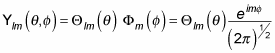##### Quantum Physics For Dummies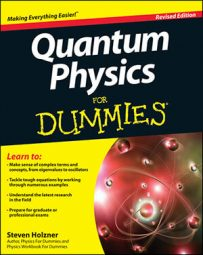At some point, your quantum physics instructor may ask you to find the eigenfunctions of Lz in spherical coordinates. In spherical coordinates, the Lz operator looks like this: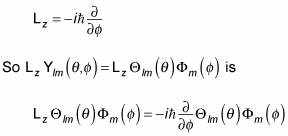which is the following: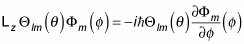And because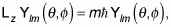this equation can be written in this version: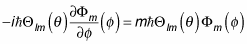Cancelling out terms from the two sides of this equation gives you this differential equation: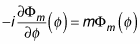This looks easy to solve, and the solution is just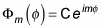where C is a constant of integration.

You can determine C by insisting that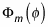be normalized — that is, that the following hold true: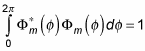(Remember that the asterisk symbol [*] means the complex conjugate. A complex conjugate flips the sign connecting the real and imaginary parts of a complex number.)

So this gives you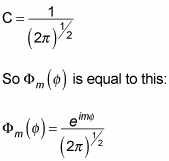You are now able to determine the form of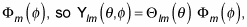which equals